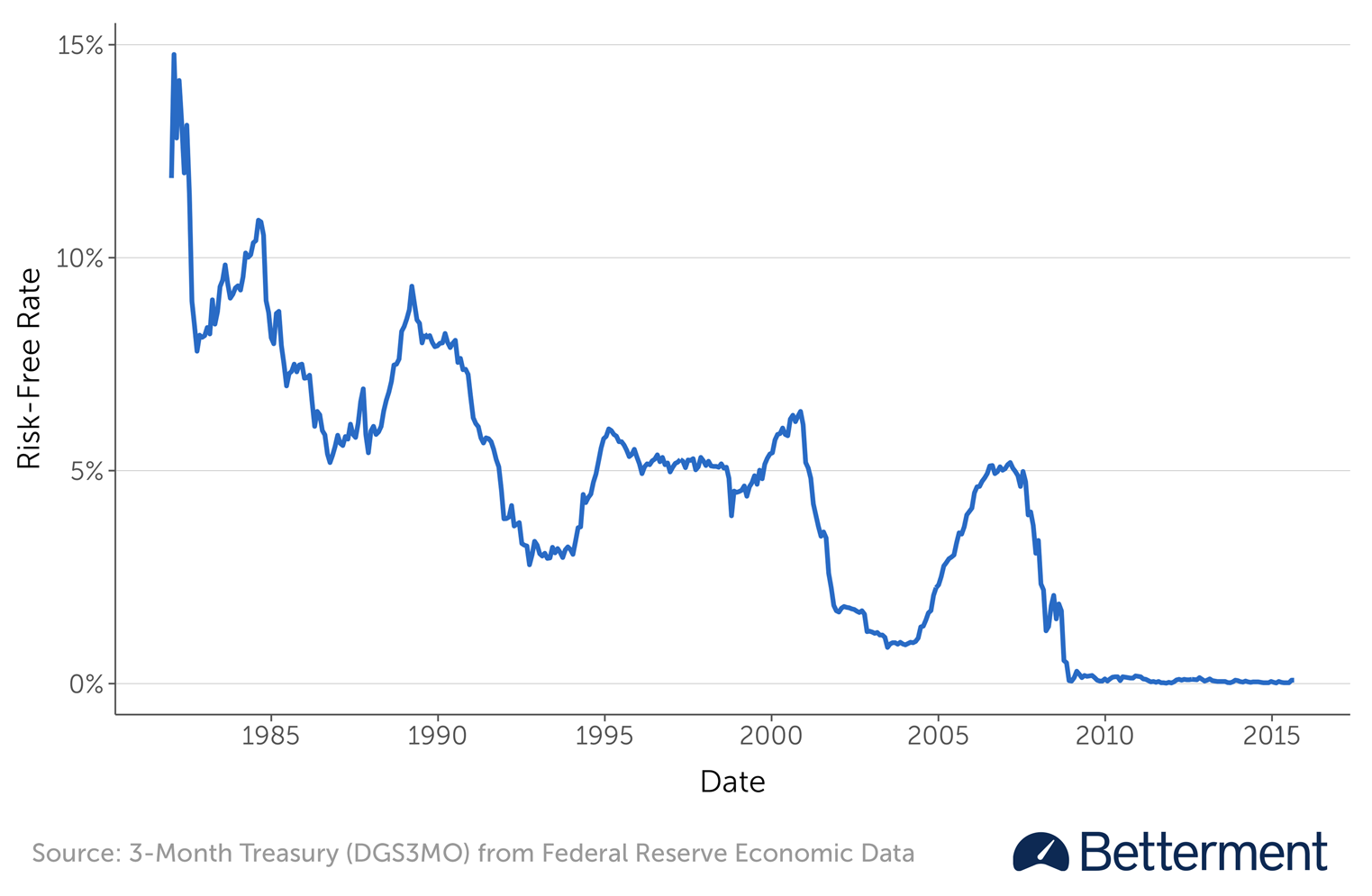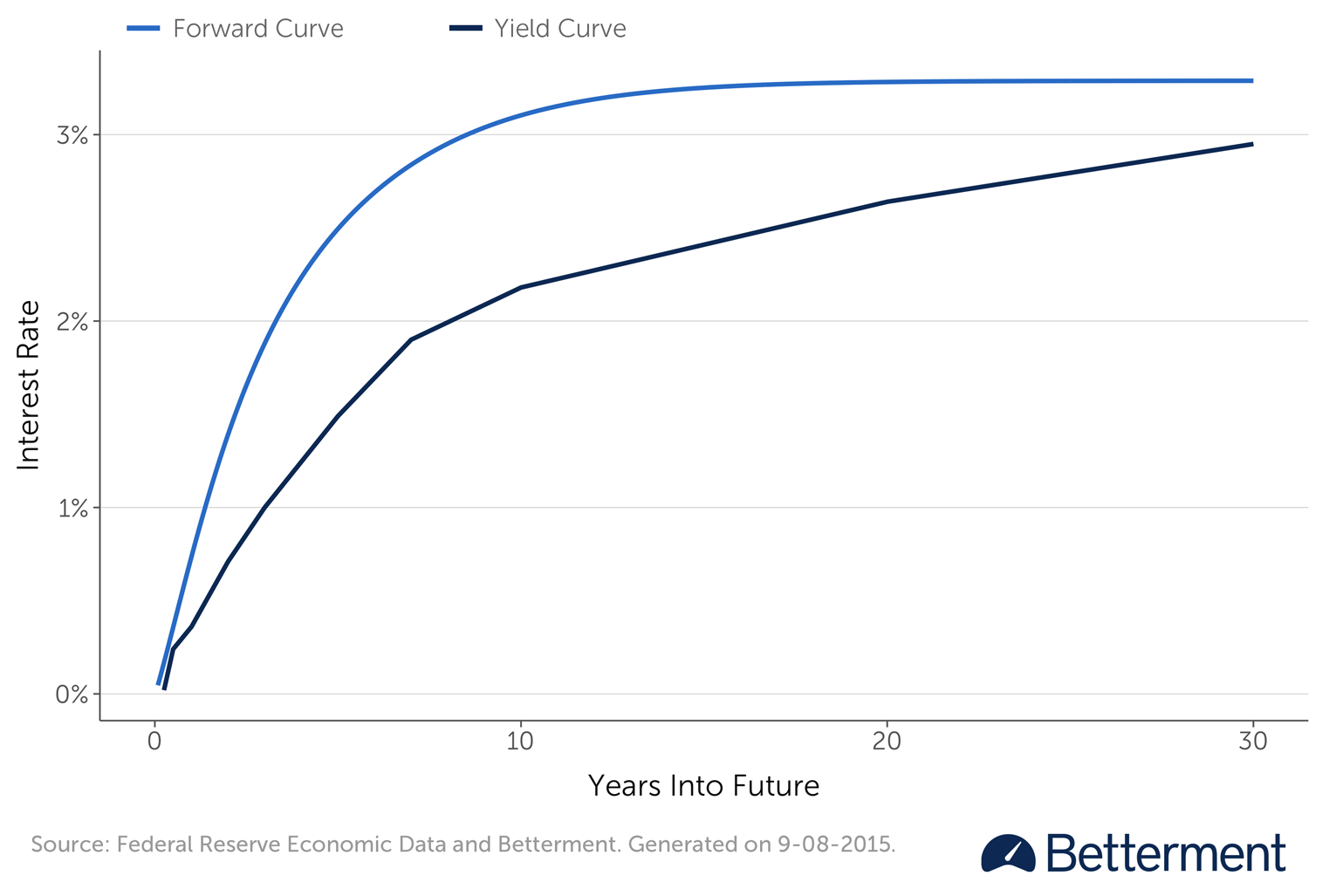# The Forward Curve: How Betterment Forecasts Interest Rates

## Does your automated investing service regularly update its forecasted future risk-free rate assumption? At Betterment, the answer is yes, on a quarterly basis.The SEC and FINRA issued an alert in May 2015 about automated investing services. In the process, they surfaced some good questions consumers should ask before placing their money with a service that uses algorithms to drive advice and portfolio management.

As transparency is one of the core principles of Betterment, we make ongoing efforts to educate investors on the assumptions that we use in our algorithms. We took this alert as an opportunity to do more of just that.

The alert specifically drew focus to interest rate assumptions underlying automated investing: “If the automated tool assumes that interest rates will remain low but, instead, interest rates rise, the tool’s output will be flawed.” This is another way of saying, “Does your automated investing service regularly update its forecasted future risk-free rate assumption?” At Betterment, the answer is yes, on a quarterly basis.

But what does that mean, and why is it significant? To answer that, we’ll need to understand the forward interest rate curve.

## The Risk-Free Rate

The risk-free rate is a rate of return for an investment with nearly zero risk—such as an ultra short-term Treasury. The fact that the issuer is the U.S. government means it has insignificant credit risk, and the ultra short-term maturity means it has minimal interest rate exposure. Of course, this ignores inflation risk, but you know exactly what your return will be, in nominal returns.

For as long as I can remember, economics and finance textbooks spoke of a risk-free rate pegged from 2% to 5%. This made classroom math easier, but for the past decade of low interest rates, the number doesn’t come close to reflecting the real world.

When we calculate returns, both historic and forward-looking, we consider a portfolio’s performance in the context of the risk-free rate in the same period. If you could have achieved the same (or higher) return risk free, why would you take the risky asset?

In the typical classroom example, if an investment earned a 12% return last year, we would want to adjust that down by the risk-free rate—let’s say 5%—which paints that investment as a seven percentage point return above the risk-free rate. This is what we call an excess return—a return above the risk-free rate. From the other perspective, if an investor wants to consider a new potential investment that takes on risk, it would need to offer an expectation of a return greater than the risk-free rate. Otherwise, why bother?

In these examples, the ultra short-term U.S. Treasury is usually the stand in for risk-free rate. While that was 5% or more for years (that was the rate in 2000), today the three-month U.S. Treasury yield is actually just 0.04%.

Risk-Free Rate Since 1982So, the first implied question in the regulatory alert item is: Does the Betterment algorithm know that the three-month U.S. Treasury yield is no longer anywhere near 5%, but a lot closer to 0.04%? The answer is yes. And, that number is automatically updated in the underlying algorithm on a quarterly basis.

## Future Risk-Free Rates: What Will They Be?

Of course, if you have a 30-year goal with Betterment, you don’t expect risk-free rates to be at zero over the next 30 years. But how might we forecast future risk-free rates, especially without resorting to overconfident expert judgments?

Well, we can use the market’s estimate. Because the bond market is very efficient, we can use market prices to estimate the expected yields at specific points in time in the future.

The classic example is a bond with a two-year maturity and a bond with a five-year maturity need to move together, so that an investor could buy the two-year instrument and then afterward invest in some three-year instrument to bring him to the total return of the five-year instrument. If the yields are known on the two-year and on the five-year bonds, calculating what the three-year note would yield becomes the question at hand.

The CFA Institute gives a nice example of how this works.

If our two-year bond is paying 2%, then the math is:

100 x (1.02) x (1.02) = 100 x (1.02)^2 = \$104.4

If our five-year bond is paying 5%, then the math equation would be similar:

100 x (1.05)^5 = \$127.63

Future forward rates refer to what is the implied rate for a bond we could hold for years three, four, and five, whose return would allow the owner of the two-year bond to have the same total return as the original five-year bond.

A simple equation to solve for the missing variable (i) would be:

\$104.04 x (1 + i)^3 = \$127.63

That means the first return, times 1 plus the missing interest rate, and that raised to the power equal to the number of years, has to equal the return from the full five-year yield.

Doing some easy algebra brings you to:

Forward interest rate = [(\$127.63/\$104.4)^⅓] - 1

In this example, i = 0.070489, or 7.05%

So, the answer is that the forward rate, implied by the two original returns, means that an investor could earn 2% on the two-year bond, then invest that in a three-year bond yielding 7.05%, to equal what he could have also got through owning the five-year bond yielding 5%. Note that the three-year bond will have a higher yield than the average yield of the five-year. Because the five-year is an average over five years, if the first two years are below average, the following years must be above average.

We can see this relationship in the actual bootstrapped forward curve below—the implied forward curve is higher than the yield curve.

Yield and Forward Curves## What This Means for You

Expected future returns are comprised of two parts—the risk premium (above the risk-free rate) that assets earn, and the risk-free rate.

Expected Return = Risk-Free Rate + Risk Premium (or excess return)

When we forecast our forward-looking returns, the risk-premium component of the portfolio changes at a gradual rate, but the risk-free component can reflect the bond market’s forecasts over the course of your goal.

For customers, what’s important is that our estimates of expected returns are being updated to take into account market forecasts of future risk-free rates. If future risk-free rates are anemic, this means you might need to save more to achieve the same goal. On the other hand, if future rates rise, it may mean you will have more certainty about having at least as much as, if not more than, your goal target. We adjust this quarterly so that our forecasts can be as accurate as possible for our clients, be their goals short term or long term. We don’t believe more frequent than quarterly would be beneficial.

While future risk-free rates are important for our savings advice, they don’t influence our allocation advice. Betterment’s investment philosophy is that you should diversify a portfolio and hold it for the long term, adjusting frequently, but not taking big bets that can easily be off. As for the original question posed by the SEC about the underlying assumptions used in the algorithm, rest assured that Betterment’s algorithm is using current and accurate data.